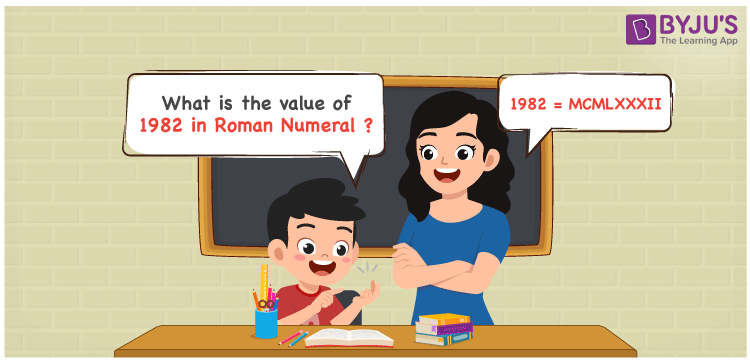# 1982 in Roman Numerals

1982 in Roman numerals is MCMLXXXII. To convert 1982 in Roman Numerals, we will write 1982 in the expanded form, i.e. 1982 = 1000 + (1000 – 100) + 50 + 10 + 10 + 10 + 1 + 1 thereafter replacing the transformed numbers with their respective roman numerals, we get 1982 = M + (M – C) + L + X + X + X + I + I = MCMLXXXII. In this article, we will explain how to correctly transform 1982 in Roman numerals.

 Number Roman Numeral 1982 MCMLXXXII## How to Write 1982 in Roman Numerals?

In order to write 1982 in Roman Numerals, it has to be written in expanded form, i.e.

1982 = 1000 + (1000 – 100) + 50 + 10 + 10 + 10 + 1 + 1

1982 = M + (M – C) + L + X + X + X + I + I

1982 = MCMLXXXII

## Video Lesson on Roman Numerals## Frequently Asked Questions on 1982 in Roman Numerals

Q1

### Write 1982 in Roman Numerals.

In Roman Numerals, 1982 is written as MCMLXXXII.
Q2

### What does 1982 in Roman Numerals Mean?

To write 1982 in roman numerals, we will first express 1982 in expanded form.
1982 = 1000 + (1000 – 100) + 50 + 10 + 10 + 10 + 1 + 1 = M + (M – C) + L + X + X + X + I + I = MCMLXXXII. Hence, 1982 in roman numbers is expressed as MCMLXXXII.
Q3

### How to convert 1982 into Roman Numerals?

The conversion of 1982 into Roman Numerals includes breaking the number according to its place value.
Thousands = 1000 = M
Hundreds = 900 = CM
Tens = 80 = LXXX
Ones = 2 = II
Number = 1000 + 900 + 80 + 2 = M + CM + LXXX + II = MCMLXXXII